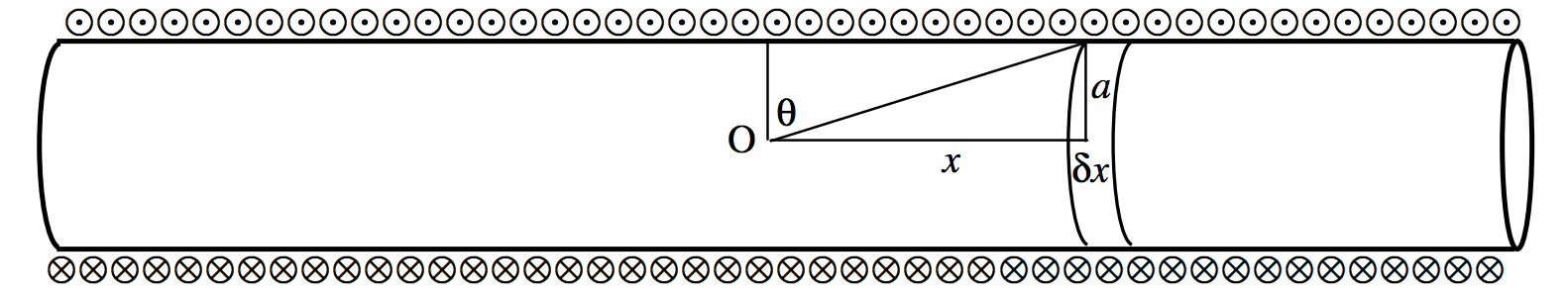$$\require{cancel}$$

# 6.8: Field on the Axis of a Long Solenoid

•• Contributed by Jeremy Tatum
• Emeritus Professor (Physics & Astronomy) at University of Victoria

The solenoid, of radius $$a$$, is wound with $$n$$ turns per unit length of a wire carrying a current in the direction indicated by the symbols $$\bigotimes$$ and $$\bigodot$$.$$\text{FIGURE VI.8}$$

At a point O on the axis of the solenoid the contribution to the magnetic field arising from an elemental ring of width $$\delta x$$ (hence having $$n\, δx$$ turns) at a distance $$x$$ from O is

$\delta B = \frac{\mu n \,\delta x\, I a^2}{2(a^2+x^2)^{3/2}}=\frac{\mu nI}{2a}\cdot \frac{a^3 \delta x}{(a^2+x^2)^{3/2}}.\label{6.8.1}$

This field is directed towards the right.

Let us express this in terms of the angle $$θ$$.

We have $$x=a \tan \theta ,\, \delta x = a \sec^2 \theta \, \delta \theta ,\text{ and }\frac{a^3}{(a^2+x^2)^{3/2}}=\cos^3 \theta$$. Equation \ref{6.8.1} becomes

$\delta B = \frac{1}{2}\mu nI\cos \theta .$

If the solenoid is of infinite length, to find the field from the entire infinite solenoid, we integrate from $$θ = \pi/2 \text{ to }0$$ and double it. Thus

$B=\mu nI \int_0^{\pi/2}\cos \theta \,d\theta.$

Thus the field on the axis of the solenoid is

$B=\mu n I.$

This is the field on the axis of the solenoid. What happens if we move away from the axis? Is the field a little greater as we move away from the axis, or is it a little less? Is the field a maximum on the axis, or a minimum? Or does the field go through a maximum, or a minimum, somewhere between the axis and the circumference? We shall answer these questions in section 6.11.